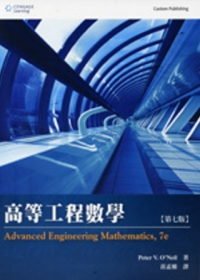{itemname} {itemname}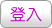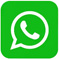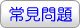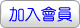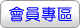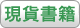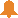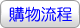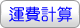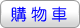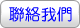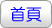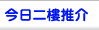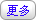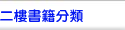Advanced Engineering Mathematics (Custom Edition) (bundle Coursemate)7/E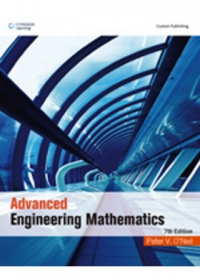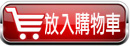沒有庫存 訂購需時10-14天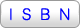9789000014620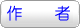Peter V. O’Neil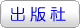俊傑書局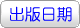2013年2月22日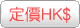427.00  元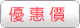HK\$ 362.95省下 \$64.05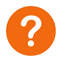[ 尚未分類 ]

Through previous editions, Peter O’Neil has made rigorous engineering mathematics topics accessible to thousands of students by emphasizing visuals, numerous examples, and interesting mathematical models. Now, ADVANCED ENGINEERING MATHEMATICS features revised examples and problems as well as newly added content that has been fine-tuned throughout to improve the clear flow of ideas. The computer plays a more prominent role than ever in generating computer graphics used to display concepts and problem sets.

In this new edition, computational assistance in the form of a self contained Maple Primer has been included to encourage students to make use of such computational tools.

Peter V. O’Neil

現職：University of Alabama, Birmingham

PART I: ORDINARY DIFFERENTIAL EQUATIONS
Ch 1 First-Order Differential Equations
Ch 2 Linear Second-Order Equations
Ch 3 The Laplace Transform
Ch 4 Series Solutions
Ch 5 Approximation Of Solutions

PART II: VECTORS, LINEAR ALGEBRA, AND SYSTEMS OF LINEAR DIFFERENTIAL EQUATIONS
Ch 6 Vectors And Vector Spaces
Ch 7 Matrices And Linear Systems
Ch 8 Determinants
Ch 9 Eigenvalues, Diagonalization And Special Matrices
Ch10 Systems Of Linear Differential Equations

PART III: VECTOR ANALYSIS
Ch11 Vector Differential Calculus
Ch12 Vector Integral Calculus

PART IV: FOURIER ANALYSIS, SPECIAL FUNCTIONS, AND EIGENFUNCTION EXPANSIONS
Ch13 Fourier Series
Ch14 The Fourier Integral And Transforms
Ch15 Special Functions And Eigenfunction Expansions

PART V: PARTIAL DIFFERENTIAL EQUATIONS
Ch16 The Wave Equation
Ch17 The Heat Equation
Ch18 The Potential Equation

PART VI: COMPLEX FUNCTIONS
Ch19 Complex Numbers And Functions
Ch20 Complex Integration
Ch21 Series Representations Of Functions
Ch22 Singularities And The Residue Theorem
Ch23 Conformal Mappings And Applications

Appendix A Maple Primer
 其 他 著 作 1. 工程數學(8版)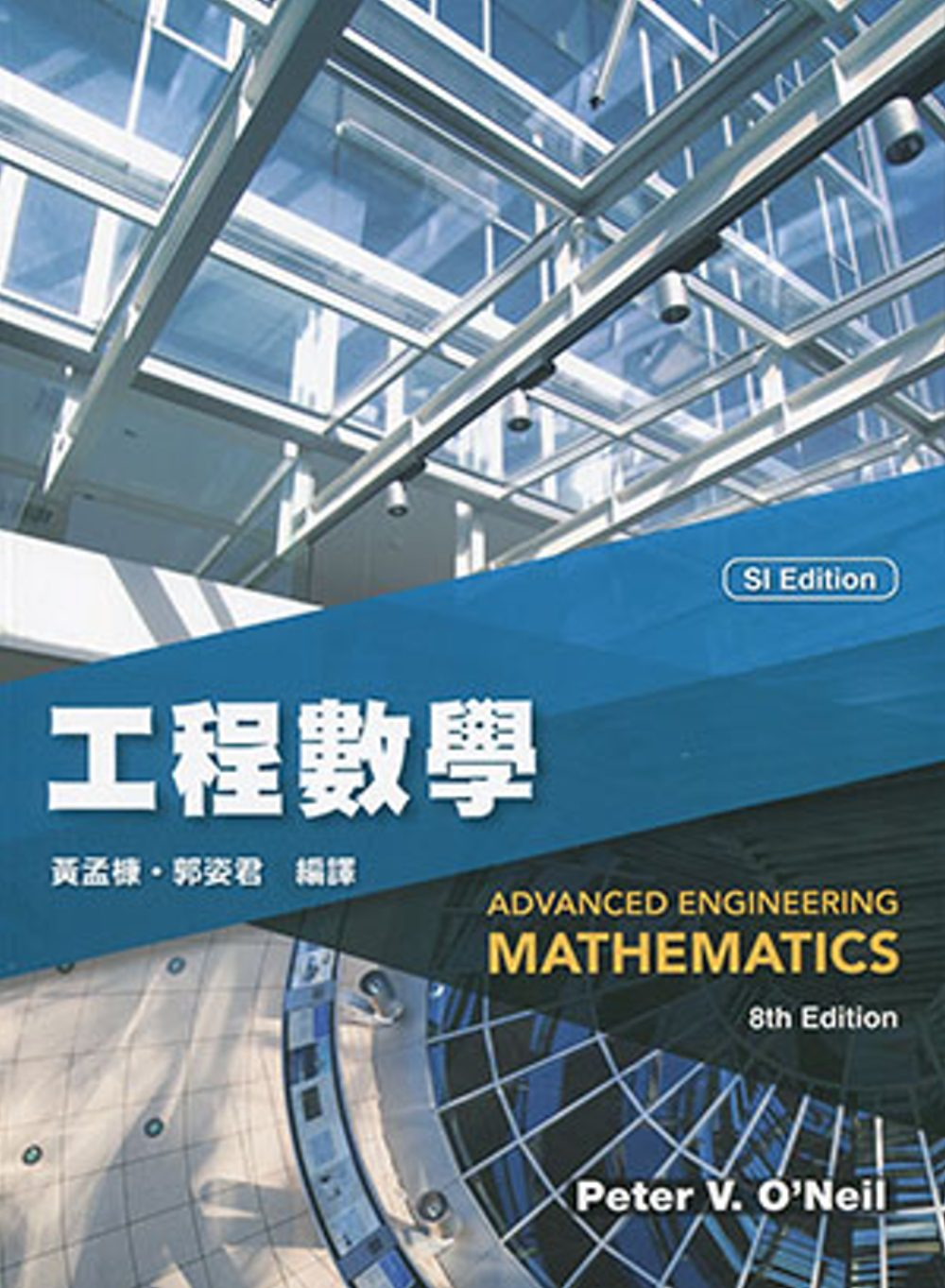2. 高等工程數學(8版)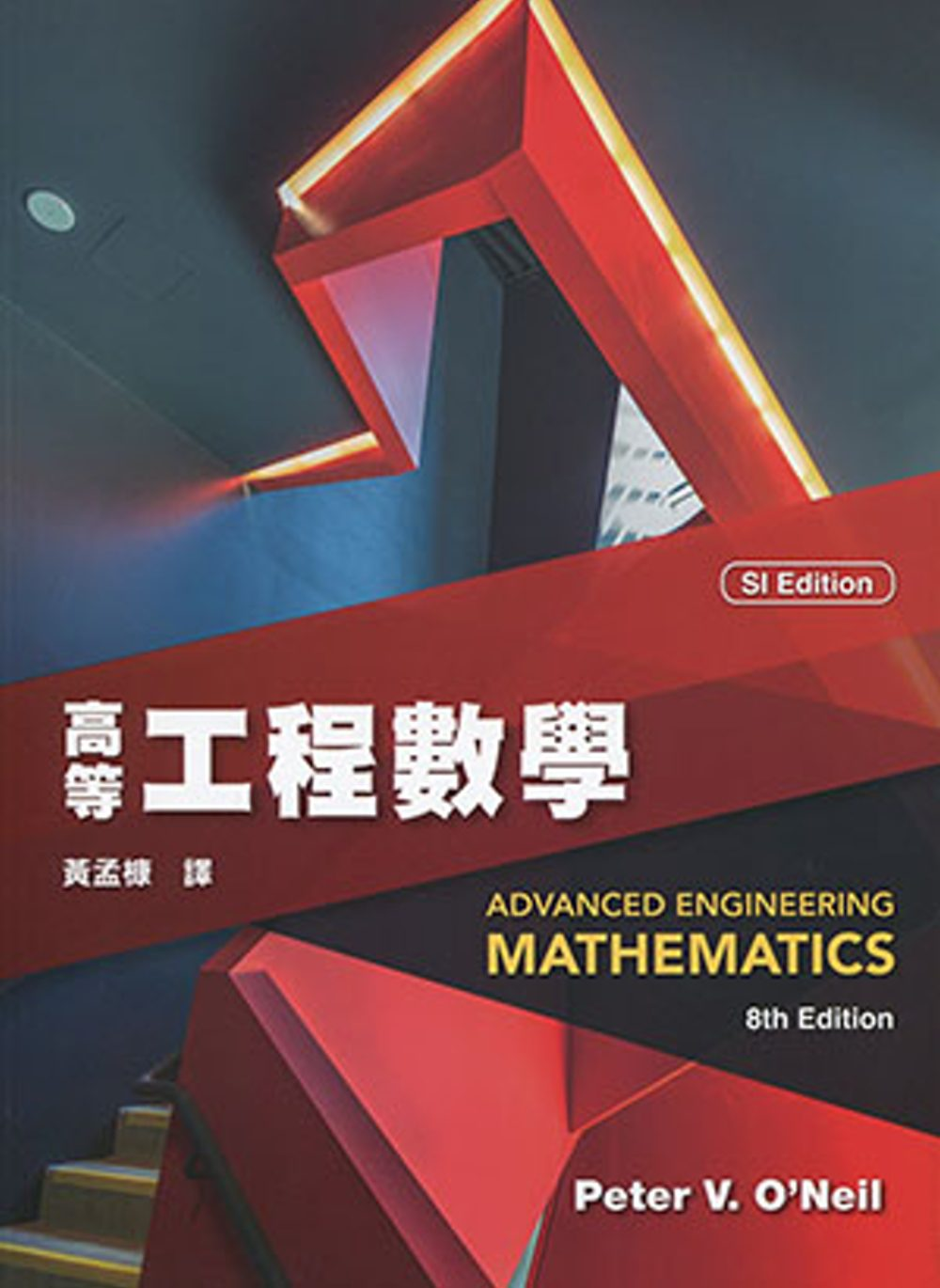3. Advanced Engineering Mathematics(SI Edition)(Custom Solutions)(8版)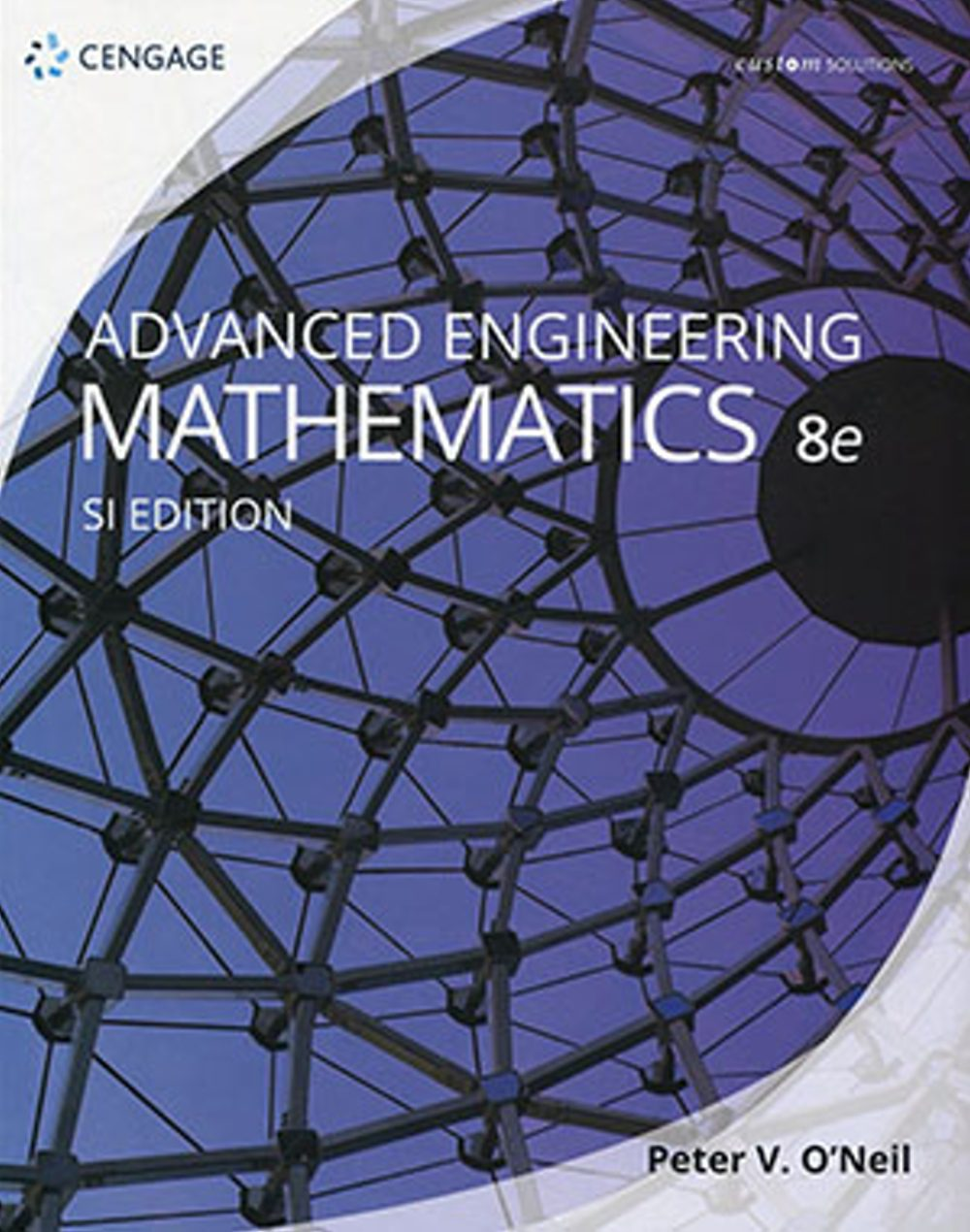4. Advanced Engineering Mathematics(SI Edition)(8版)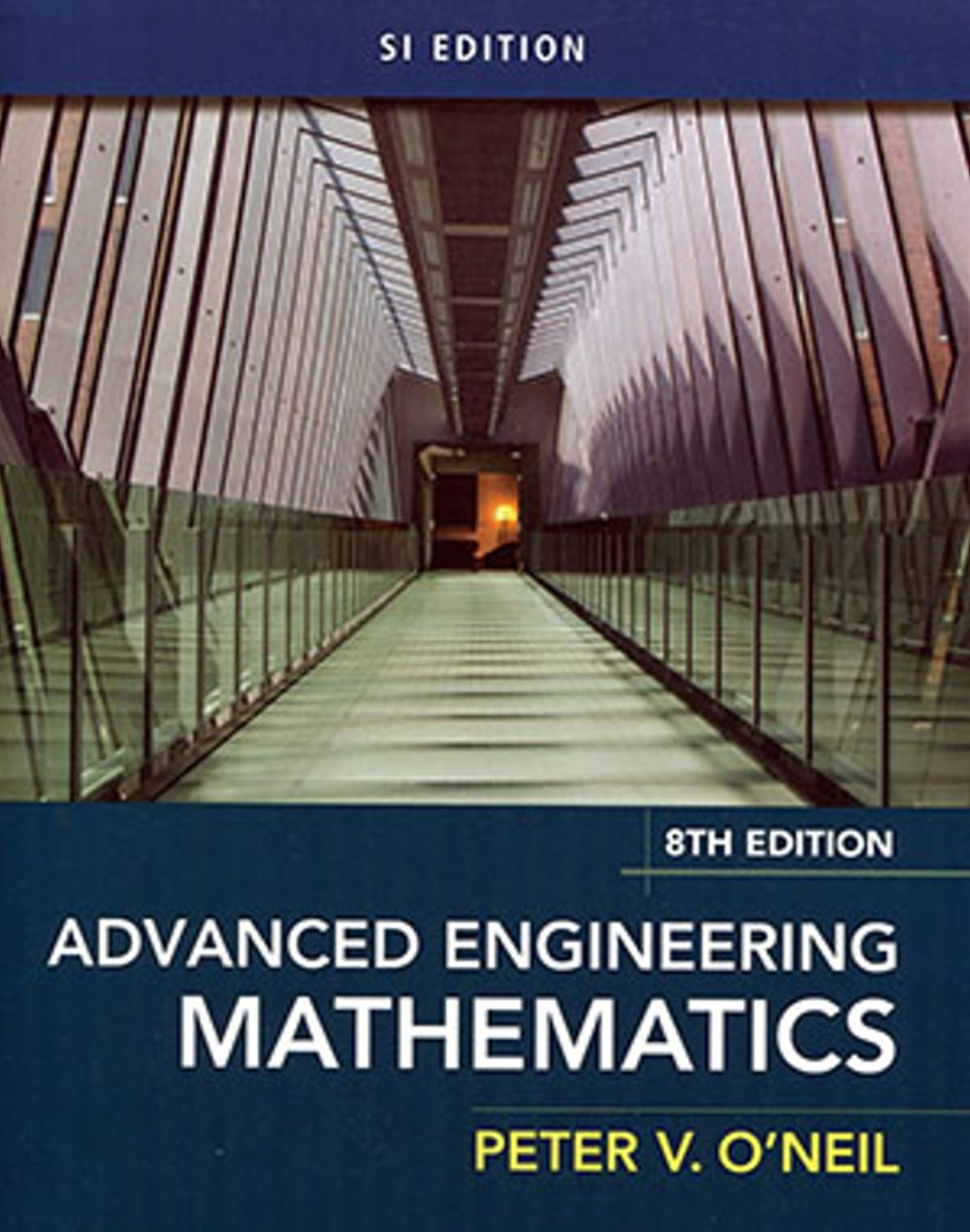5. 高等工程數學(7版) O’Neil/ Advanced Engineering Mathematics 7/e